منتدى كلية العلوم بدمنهور هل تريد التفاعل مع هذه المساهمة؟ كل ما عليك هو إنشاء حساب جديد ببضع خطوات أو تسجيل الدخول للمتابعة.منتدى كلية العلوم بدمنهور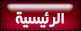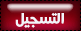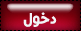منتدى كلية العلوم بدمنهور :: اخبار واقسام الكليه News and departments of the faculty :: علم الكيمياء

# Resonance"كاتب الموضوعرسالة
smart way
المدير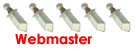mms :اوسمه :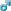عدد الرسائل : 4366
العمر : 29
العمل/الترفيه : معيد بكليه العلوم جامعه الاسكندريه
المزاج : nice
جنسيتك : آخر....
السٌّمعَة : 25
تاريخ التسجيل : 22/03/2009موضوع: Resonance"الإثنين نوفمبر 02, 2009 5:27 am

Kekulé
structural formulas are essential tools for understanding organic
chemistry. However, the structures of some compounds and ions cannot be
represented by a single formula. For example, sulfur dioxide (SO2) and nitric acid (HNO3)
may each be described by two equivalent formulas (equations 1 & 2).
For clarity the two ambiguous bonds to oxygen are given different
colors in these formulas.

 1) sulfur dioxide2) nitric acid

If
only one formula for sulfur dioxide was correct and accurate, then the
double bond to oxygen would be shorter and stronger than the single
bond. Since experimental evidence indicates that this molecule is bent
(bond angle 120&ordm;) and has equal length sulfur : oxygen bonds
(1.432 &Aring;), a single formula is inadequate, and the actual
structure resembles an average of the two formulas. This averaging of
electron distribution over two or more hypothetical contributing
structures (canonical forms) to produce a hybrid electronic
structure is called resonance. Likewise, the structure of nitric acid
is best described as a resonance hybrid of two structures, the double
headed arrow being the unique symbol for resonance.
The above
examples represent one extreme in the application of resonance. Here,
two structurally and energetically equivalent electronic structures for
a stable compound can be written, but no single structure provides an
accurate or even an adequate representation of the true molecule. In
cases such as these, the electron delocalization described by resonance
enhances the stability of the molecules, and compounds or ions composed
of such molecules often show exceptional stability.

 3) formaldehydeThe
electronic structures of most covalent compounds do not suffer the
inadequacy noted above. Thus, completely satisfactory Kekulé formulas
may be drawn for water (H2O), methane (CH4) and acetylene C2H2).
Nevertheless, the principles of resonance are very useful in
rationalizing the chemical behavior of many such compounds. For
example, the carbonyl group of formaldehyde (the carbon-oxygen double
reactions can be explained by a small contribution of a dipolar
resonance contributor, as shown in equation 3. Here, the first
contributor (on the left) is clearly the best representation of this
molecular unit, since there is no charge separation and both the carbon
and oxygen atoms have achieved valence shell neon-like configurations
by covalent electron sharing. If the double bond is broken
heterolytically, formal charge pairs result, as shown in the other two
structures. The preferred charge distribution will have the positive
charge on the less electronegative atom (carbon) and the negative
charge on the more electronegative atom (oxygen). Therefore the middle
formula represents a more reasonable and stable structure than the one
on the right. The application of resonance to this case requires a
weighted averaging of these canonical structures. The double bonded
structure is regarded as the major contributor, the middle structure a
minor contributor and the right hand structure a non-contributor. Since
the middle, charge-separated contributor has an electron deficient
carbon atom, this explains the tendency of electron donors
(nucleophiles) to bond at this site.
The basic principles of the resonance method may now be summarized.
For
a given compound, a set of Lewis / Kekulé structures are written,
keeping the relative positions of all the component atoms the same.
These are the canonical forms to be considered, and all must have the
same number of paired and unpaired electrons.

The following
factors are important in evaluating the contribution each of these
canonical structures makes to the actual molecule.

The number of covalent bonds in a structure. (The greater the bonding, the more important and stable the contributing structure.)

Formal charge separation. (Other factors aside, charge separation decreases the stability and importance of the contributing structure.)

Electronegativity of charge bearing atoms and charge density.
(High charge density is destabilizing. Positive charge is best
accommodated on atoms of low electronegativity, and negative charge on
high electronegative atoms.)

The stability of a resonance hybrid is always greater than the stability of any canonical contributor.
Consequently, if one canonical form has a much greater stability than
all others, the hybrid will closely resemble it electronically and
energetically. This is the case for the carbonyl group (eq.3). The left
hand C=O structure has much greater total bonding than either
charge-separated structure, so it describes this functional group
rather well. On the other hand, if two or more canonical forms have
identical low energy structures, the resonance hybrid will have
exceptional stabilization and unique properties. This is the case for
sulfur dioxide (eq.1) and nitric acid (eq.2).

 4) carbon monoxide5) azide anion

To
illustrate these principles we shall consider carbon monoxide (eq.4)
and azide anion (eq.5). In each case the most stable canonical form is
on the left. For carbon monoxide, the additional bonding is more
important than charge separation. Furthermore, the double bonded
structure has an electron deficient carbon atom (valence shell sextet).
A similar destabilizing factor is present in the two azide canonical
forms on the top row of the bracket (three bonds vs. four bonds in the
left most structure). The bottom row pair of structures have four
bonds, but are destabilized by the high charge density on a single
nitrogen atom.
All the examples on this page demonstrate an important restriction that must be remembered when using resonance.No atoms change their positions within the common structural framework. Only electrons are moved.

_________________

I am Afraid of Being Happy
Cuz Every Time I am Happy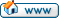Resonance"